Vibroengineering PROCEDIA

Published: 19 October 2018

# Research of multi-concurrent fault diagnosis of rotating machinery based on VMD and KICA

Hongchun Sun1
Liang Fang2
1, 2School of Mechanical Engineering and Automation, Northeastern University, Shenyang, China
1, 2Key Laboratory of Vibration and Control of Aero-Propulsion Systems of Ministry of Education, Northeastern University, Shenyang, China
Corresponding Author:
Hongchun Sun
Views 27

#### Abstract

In order to improve the multi-concurrent fault diagnosis of rotating machinery, a feature extraction method based on variational mode decomposition (VMD) and kernel independent component analysis (KICA) is proposed. First, use VMD to improve the dimension of single-channel vibration signal. Then, calculate the correlation coefficient between the signal of each dimension and the original signal. Finally, high correlation signals are used to form a new observation signal and the fault signals will be extracted by KICA. Compared with ensemble empirical mode decomposition (EEMD) + fast independent component analysis (FastICA), the better performance of the proposed method is demonstrated by an analysis of rolling bearing with the fault of inner ring and outer ring mixed. Furthermore, an experiment with the fault of outer ring of rolling bearing and gear breaking mixed verifies the effectiveness of this method. The result demonstrates that the proposed method is efficient for fault diagnosis of single-channel vibration signal of rotating machinery with multi-concurrent faults.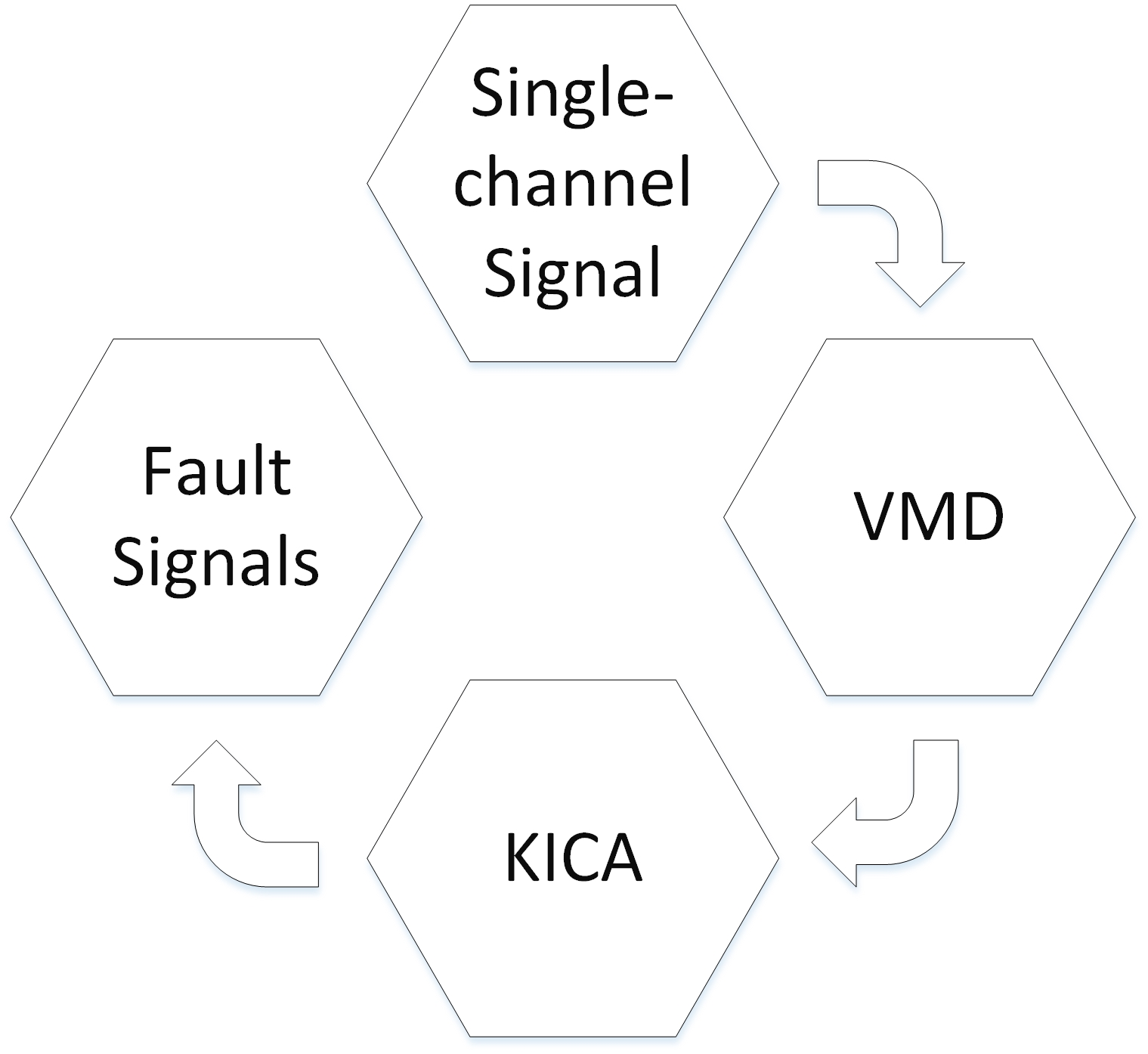#### Highlights

• A method of combining VMD with KICA is proposed.
• The goal is to diagnose multi-concurrent faults of rotating machinery.
• Experiments demonstrate that this method can diagnose the fault of complex rotating machinery.

## 1. Introduction

With the rapid development of modern industry, rotating machinery is becoming more and more large-scale, high-speed and complex. Fault diagnosis technology is playing an increasingly important role in ensuring the safe, reliable and efficient operation of equipment. However, due to the complexity of the structure and technology of rotating machinery, multi-concurrent faults often occur, which brings great difficulties to diagnosis. Nowadays, in the field of fault diagnosis, one of the most common methods is EEMD + FastICA [1, 2]. EEMD [3, 4] is widely used in fault feature extraction, but it sometimes has mode mixing. Moreover, it is affected by the sampling frequency and the decomposition error sometimes is large. In order to avoid these problems, a new adaptive signal processing method, VMD , is proposed. This method is used to search the optimal solution of the variational model by iterative search to determine the frequency center and bandwidth of each decomposition component. FastICA [6, 7] and KICA [8, 9] which are blind source separation algorithms are often used in fault diagnosis recently. Compared with FastICA, KICA has the advantage of dealing with nonlinear signals. The real signals of rotating machinery usually contain nonlinear characteristics. Therefore, this paper proposes a fault diagnosis method combining the merits of VMD and KICA.

## 2. Theory

### 2.1. The proposed method

In this paper, a fault diagnosis method of rotating machinery based on VMD-based KICA is proposed. The procedure of the proposed method has been summarized in Fig. 1. The detailed procedure is described as follows:

Step 1: The collected single-channel signal $\mathbf{X}\left(t\right)$ is decomposed with VMD and each VM can be obtained.

Step 2: Calculate the correlation coefficient of each VM to $\mathbf{X}\left(t\right)$. Then, choose high correlation VMs to form a new observation signal.

Step 3: Use KICA to extract the fault signals from the new observation signal.

Fig. 1The summarized procedure of the proposed method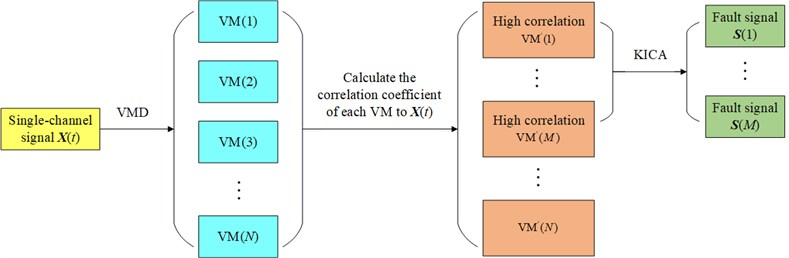### 2.2. Evaluation index $\mathbf{\rho }$

The fault signal extracted from the real signal generally contains some noise. In the power spectrum of the extracted fault signal, the power of the fault frequency is usually the highest, and other frequencies can be considered as noise. If the power of the noise is very close to or even higher than the power of the fault frequency, the fault type cannot be accurately determined. Therefore, in order to compare the extraction effect of each fault diagnosis method, this paper proposes an evaluation index $\rho$:

1
$\rho =\frac{{P}_{f}-{P}_{s}}{{P}_{s}},$

where ${P}_{f}$ is the power of fault frequency, ${P}_{s}$ is the power of secondary high frequency. Therefore, the bigger the value of $\rho$, the better the effect of the extraction.

## 3. Experiment

### 3.1. Fault of inner ring and outer ring of rolling bearing mixed

The experimental device is shown in Fig. 2. The whole device is driven by a 550 W (220 V-50 Hz) AC motor and drives the shaft system with couplings. The shaft section between two bearing seats is equipped with a belt wheel, and the belt drives the active gear shaft of gear box. There are two rolling bearings on the shaft system. The inner and outer rings of the rolling bearing are respectively machined with tiny pitting pits of 0.3 mm in depth and 0.5 mm in diameter to simulate the fault of inner ring and outer ring. One acceleration transducer is installed vertically on the shell near the rolling bearing. The sampling frequency ${f}_{s}=$ 8192 Hz and sampling point number is 8192. The motor speed is 850 rpm, that is, the rotation frequency ${f}_{r}=$ 14.2 Hz.

The equation of fault frequency of inner ring is:

2
${f}_{ic}=\frac{1}{2}N\left(1+\frac{d}{D}\mathrm{c}\mathrm{o}\mathrm{s}\alpha \right){f}_{r}.$

The equation of fault frequency of outer ring is:

3
${f}_{oc}=\frac{1}{2}N\left(1-\frac{d}{D}\mathrm{c}\mathrm{o}\mathrm{s}\alpha \right){f}_{r},$

where $N$ is the number of roller, $d$ is the diameter of roller, $D$ is the pitch diameter of bearing, and $\alpha$ is bearing contact angle. According to Eq. (2) and Eq. (3), ${f}_{ic}=$ 106 Hz and ${f}_{oc}=$ 70 Hz.

Fig. 2The experimental device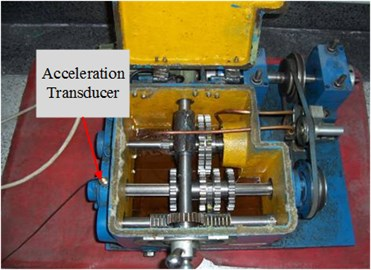### 3.1.1. EEMD + FastICA

In this method, the mixed signal $x$ is decomposed by EEMD first and each intrinsic mode function (IMF) can be obtained. Then, calculate the correlation coefficient of each IMF to $x$. IMFs with high correlation coefficient were selected to reconstruct the new observation signal. Finally, the fault signals can be extracted by FastICA. The power spectrum of the extracted fault signals is shown in Fig. 3.

Fig. 3The power spectrum of the extracted fault signals based on EEMD + FastICA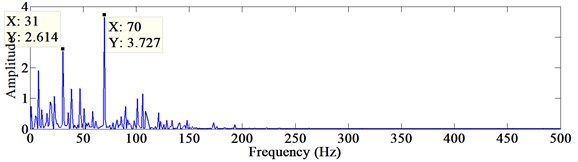a)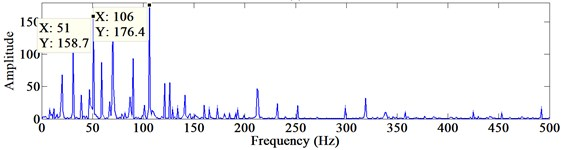b)

The frequency of 70 Hz can be seen in Fig. 3(a), and the frequency of 106 Hz can be seen in Fig. 3(b). According to Eq. (1), ${\rho }_{oc}=$ 0.426, ${\rho }_{ic}=$ 0.112. However, in Fig. 3(b), the amplitude of the frequency of 70 Hz is very large. Mode mixing has a great impact on fault diagnosis.

### 3.1.2. The proposed method

In this method, the mixed signal $x$ is decomposed by VMD first and each VM can be obtained. Then, calculate the correlation coefficient of each VM to $x$. VMs with high correlation coefficient were selected to reconstruct the new observed signal. Finally, the fault signals can be extracted by KICA. The power spectrum of the extracted fault signals is shown in Fig. 4.

The frequency of 70 Hz can be seen in Fig. 4(a), and the frequency of 106 Hz can be seen in Fig. 4(b). According to Eq. (1), ${\rho }_{oc}=$ 0.922, ${\rho }_{ic}=$ 1.319.

Fig. 4The power spectrum of the extracted fault signals based on VMD+KICA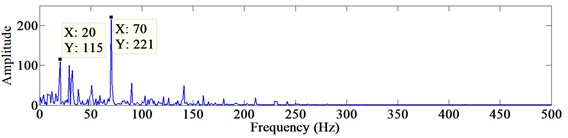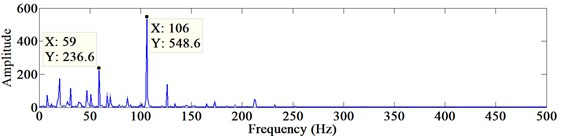The evaluation index of two methods are in Table 1. The $\rho$ of the proposed method is much bigger than EEMD + FastICA. Therefore, the proposed method has a better effect of extraction. The result proves that the proposed method is efficient to extract the features of single-channel signal with multi-concurrent faults.

Table 1Evaluation index statistics

 ${\rho }_{oc}$ ${\rho }_{ic}$ EEMD + FastICA 0.426 0.112 The proposed method 0.922 1.319

### 3.2. Fault of outer ring of rolling bearing and gear breaking mixed

In order to verify the effectiveness of fault diagnosis with two components of rotating machinery, the fault of outer ring of rolling bearing and gear breaking are applied here. The sampling frequency ${f}_{s}=$ 8192 Hz and sampling point number is 8192. The motor speed is 850 rpm, that is, the rotation frequency ${f}_{r}=$ 14.2 Hz. The number of gear teeth $Z=$ 20. The number of the broken tooth ${Z}_{b}=$ 1. According to ${f}_{c}=Z×{f}_{r}$, the gear mesh frequency ${f}_{c}=$ 283.3 Hz. When the gear is affected by broken tooth fault, the rotation frequency ${f}_{r}$ and its frequency multiplication will be the main features in frequency-domain, and the frequency ${f}_{z}={Z}_{b}×{f}_{c}$ will also exist.

Fig. 5The power spectrum of the extracted fault signals based on VMD + KICA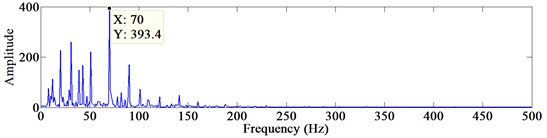a)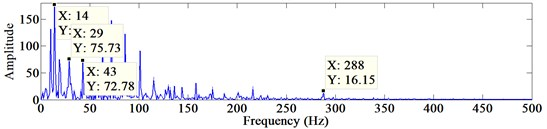b)

In the proposed method, the mixed signal $x$ is decomposed by VMD first and each VM can be obtained. Then, calculate the correlation coefficient of each VM to $x$. VMs with high correlation coefficient were selected to reconstruct the new observed signal. Finally, the fault signals can be extracted by KICA. The power spectrum of the extracted fault signals is shown in Fig. 5.

As can be seen from Fig. 5, Fig. 5(a) is the fault signal of outer ring of rolling bearing. Fig. 5(b) is the fault signal of gear breaking. Furthermore, the fault features are significantly obvious. It demonstrates that the proposed method has a good performance of fault diagnosis with the fault of outer ring of rolling bearing and gear breaking mixed.

## 4. Conclusions

The method of VMD-based KICA has been proposed to improve the multi-concurrent fault diagnosis of rotating machinery in this paper. In the experiment of the fault of inner ring and outer ring of rolling bearing mixed, evaluation index $\rho$ proves that the proposed method makes the fault features more obvious. The experiment with the fault of outer ring of rolling bearing and gear breaking mixed verifies the effectiveness of this method. Therefore, the proposed method is efficient for fault diagnosis of single-channel vibration signal of rotating machinery with multi-concurrent faults.

#### Acknowledgements

This research is subsidized by the Natural Science Foundation of China “Research on reliability theory and method of total fatigue life for large complex mechanical structures” (Grant No. U1708255).